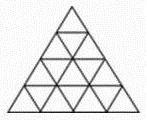## Recommended Posts

I know most of you have seen this problem at one point in time or another. The question normally wants you to count all of the triangles you see in the image. This challenge is not concerned with just counting triangles, but wants the equation which predicts the total number of triangles given $n$ number of rows.The equation uses elementary operators and I will not accept a solution that is based on floor / ceiling, modular arithmetic, or piecewise functions / two part solutions. You must provide a single function which predicts the count of triangles given any number of rows. As with all of my challenges, I will give reputation to the person who can solve this problem before I post the solution within a couple of weeks : )

Sorry for all the edits to this post. My initial search on the internet did not reveal a solution to this problem. However, subsequent searches did yield a few solutions. Luckily, I did not find the solution that I have derived and I have modified the rules to narrow down the solution I am looking for. Most of my challenges were solved many years ago, before I had a computer. Back then, I worked most of these problems out by hand or with a TI-88.

Edited by Daedalus

##### Share on other sites

Two parts to this:

Part 1: Right-side-up triangles:

In general, you will find that if there are n rows of triangles, then there are (n-m+1)(n-m+2)/2 right-side-up triangles of size m.

Upside-down triangles:

It turns out that this is effectively equal to right-side-up triangles, but only once you decrease the size of the triangle by m. So you get (n - 2m + 1) (n - 2m + 2)/2.

So we have:

$\frac{1}{2}(\sum_{m = 1}^n (n - m + 1)(n - m + 2) + \sum_{m = 1}^{m \leq \frac{n}{2}} (n - 2m + 1)(n - 2m + 2))$

There are then two cases: n even or n odd.

First: n even:

$= \frac{1}{2}(\sum_{m' = 1}^{m' = n} m'(m'+1) + \sum_{m = 1}^\frac{n}{2}(n - 2m + 1)(n - 2m + 2))$

$= \frac{1}{2}(\frac{1}{3} n(n+1)(n+2) + \sum_{m' = 0}^{\frac{n}{2} - 1} (2m' + 1)(2m' + 2))$

$= \frac{n(n + 1)(n + 2)}{6} + \sum_{m' = 0}^{\frac{n}{2} - 1} 2 m' (m' + 1) + m' + 1$

$= \frac{n(n + 1)(n + 2)}{6} + \frac{2(\frac{n}{2} - 1)\frac{n}{2}(\frac{n}{2} + 1)}{3} + \frac{(\frac{n}{2} - 1)\frac{n}{2}}{2} + \frac{n}{2}$

$= \frac{n(n + 1)(n + 2)}{6} + \frac{(n - 2)n(n + 2)}{12} + \frac{(n - 2)n}{8} + \frac{n}{2}$

$= \frac{4n^3 + 12n^2 + 8n + 2n^3 - 8n + 3n^2 - 6n + 12n}{24}$

$= \frac{2n^3 + 5n^2 + 2n}{8}$

Second: n odd:

$= \frac{1}{2}(\sum_{m' = 1}^{m' = n} m'(m'+1) + \sum_{m = 1}^\frac{n-1}{2}(n - 2m + 1)(n - 2m + 2))$

$= \frac{n(n+1)(n+2)}{6} + \frac{1}{2}\sum_1^\frac{n-1}{2}2m'(2m' + 1)$

$= \frac{n(n+1)(n+2)}{6} +\sum_1^\frac{n-1}{2}2m'(m'+1) - m'$

$= \frac{n(n+1)(n+2)}{6} + \frac{2\frac{n-1}{2}(\frac{n-1}{2} + 1)(\frac{n-1}{2} + 2)}{3} - \frac{\frac{n-1}{2}(\frac{n-1}{2} + 1)}{2}$

$= \frac{n(n+1)(n+2)}{6} + \frac{(n-1)(n+1)(n+3)}{12} - \frac{(n-1)(n+1)}{8}$

$= \frac{4n^3 + 12n^2 + 8n + 2n^3 + 6n^2 - 2n - 6 - 3n^2 + 3}{24}$

$= \frac{2n^3 + 5n^2 + 2n - 1}{8}$

So more generally, the answer is

$\frac{2n^3 + 5n^2 + 2n}{8} + \frac{(-1)^n - 1}{16}$

=Uncool-

Edited by uncool

##### Share on other sites

You are unstoppable uncool. Good job!!! I see I'm going to have to come up with an even harder challenge for you. Now let's see you crack part 2 of my second challenge : )

## Create an account

Register a new account